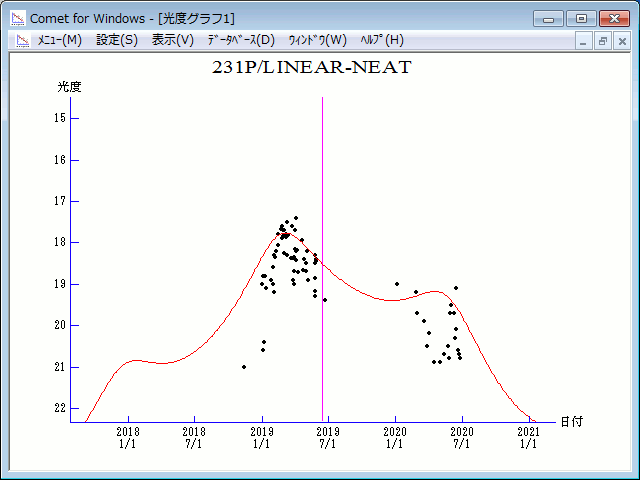# \$B%j%K%"(B-\$B%K!<%HWB@1(B

231P/LINEAR-NEAT (2019)###\$B###\$B50F;MWAG(B

```Epoch 2019 June 6.0 TT = JDT 2458640.5
T 2019 June 14.24888 TT                                 MPCW
q   3.0224784            (2000.0)            P               Q
n   0.12241839     Peri.   42.75014     -0.98583558     -0.06119911
a   4.0170287      Node   133.02971     +0.01964780     -0.96677827
e   0.2475836      Incl.   12.33409     +0.16655980     -0.24818227
P   8.05
From 456 observations 2003 Feb. 1-2019 May 5, mean residual 0".7.
Nongravitational parameters A1 = +16.22, A2 = -0.7136.
```

###\$B8wEYJQ2=(B

```        m1 = 4.3 + 5 log\$B&\$(B + 25 log r
```##### \$B50F;MWAG\$O(BM.P.E.C. 2019-J142\$B\$K7G:\\$5\$l\$?\$b\$N\$G\$9!#(B \$B8wEY%0%i%U\$O(BComet for Windows\$B\$G:n@.\$7\$?\$b\$N\$G\$9!#(B